﻿ How to Calculate the Base Current of a Transistor# How to Calculate the Base Current, IB, of a TransistorThe base current, IB, of a transistor is a crucial current of a bipolar junction transistor. Without this base current, the transistor can't turn on.

There are several ways to find the base current, IB, of a transistor. And it all depends on what information is already known about the transistor:

### 1st Way To Calculate Base Current IB

DC Analysis

Doing DC analysis of the transistor circuit is the most common way of finding out the value of IB in the circuit.The equation to solve for Ie is: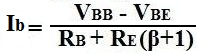So we must solve for Vbb and RB in order to solve for IB.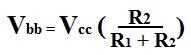Next we compute the value of RB:Now we can calculate the value of the base current, IB, in the circuit:Example

Using the example of the values for the resistors and the voltage, Vcc, above, we're going to solve for Ieq.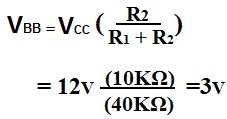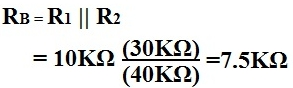### 2nd Way to Calculate Base Current IB

Using Known Values
If the emitter current, Ie, and β are known for the transistor circuit, IB can be calculated by the formula:Example
If Ie=6ma and β=99, then### 3rd Way to Calculate Base Current IB

Using Known Values
If the emitter current, Ie, and the collector current, Ic, are known, IB can be calculated by the formula: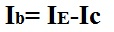Example
If Ie=4ma and Ic=3.96ma, then IB calculates out to be: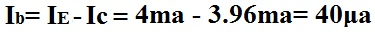Related Resources